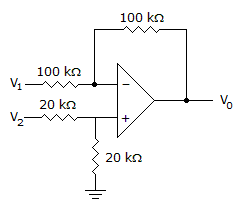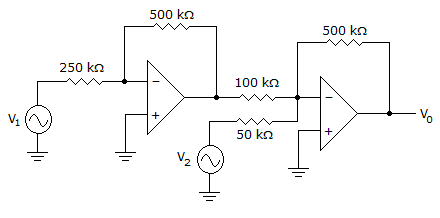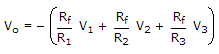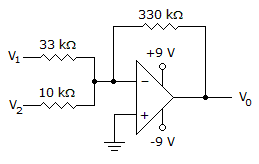# Electronic Devices - Op-Amp Applications

Exercise : Op-Amp Applications - General Questions
31.
Determine the output voltage when V1 = –V2 = –1 V.0 V
–2 V
1 V
2 V
Explanation:
No answer description is available. Let's discuss.

32.
An example of an instrumentation circuit is a(n) ________.
dc voltmeter
display driver
ac voltmeter
All of the above
Explanation:
No answer description is available. Let's discuss.

33.
Determine the output voltage.10(V2 – Vi)
–10(V2 – V1)
–10(V1 – V2)
None of the above
Explanation:
No answer description is available. Let's discuss.

34.
How many op-amps are required to implement this equation?2
3
4
1
Explanation:
No answer description is available. Let's discuss.

35.
Calculate the output voltage if V1 = 33 mV and V2 = 2 mV.0 V
–6.6 V
–0.4 V
2 V